# Python Matplotlib drawing histogram

2022-01-31 14:31:45This is my participation 11 The fourth of the yuegengwen challenge 13 God , Check out the activity details ：2021 One last more challenge

# Review

After facing matplotlib Modules from the underlying architecture 、 Basic drawing steps, etc , We have learned the line chart 、 Drawing method of histogram .

When analyzing data , We will select the corresponding chart according to the characteristics of the data to show , We need to express the concept of quality , Histogram is required .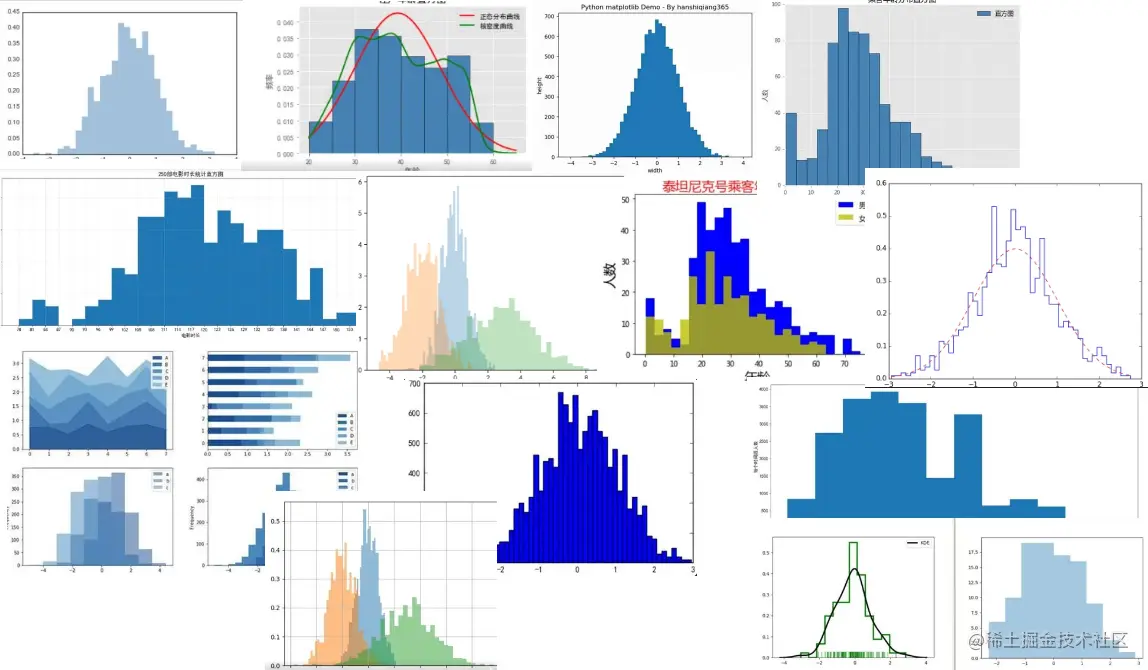In this issue , We will learn matplotlib Module draws histogram related attributes and methods ,Let‘s go~

# 1. Histogram Overview

• ## What is histogram ？

• Histogram is a visual representation of the distribution of data in continuous intervals or specific time periods
• Histogram is also called mass distribution diagram , It belongs to a kind of bar graph
• Histogram x The axis represents the data type , The vertical axis shows the distribution , Each data width can be changed arbitrarily
• ## Histogram usage scenario

• Histogram is used for probability distribution , Show the probability of occurrence of a set of data within a specified range
• It can be used to show the frequency of data distribution
• For mode 、 The position of the median
• Focus on data gaps or outliers
• ## Histogram drawing steps

1. Import matplotlib.pyplot modular
3. call pyplot.hist() Draw a histogram
• The case shows

In this case, let's analyze the rise distribution of the company's employees

• Case data preparation , Use numpy Random generation 200 An elevated data

``````import numpy as np

x_value = np.random.randint(140,180,200)
Copy code ``````
• Draw histogram

``````import matplotlib.pyplot as plt

plt.hist(x_value,bins=10)

plt.title("data analyze")
plt.xlabel("height")
plt.ylabel("rate")

plt.show()
Copy code ``````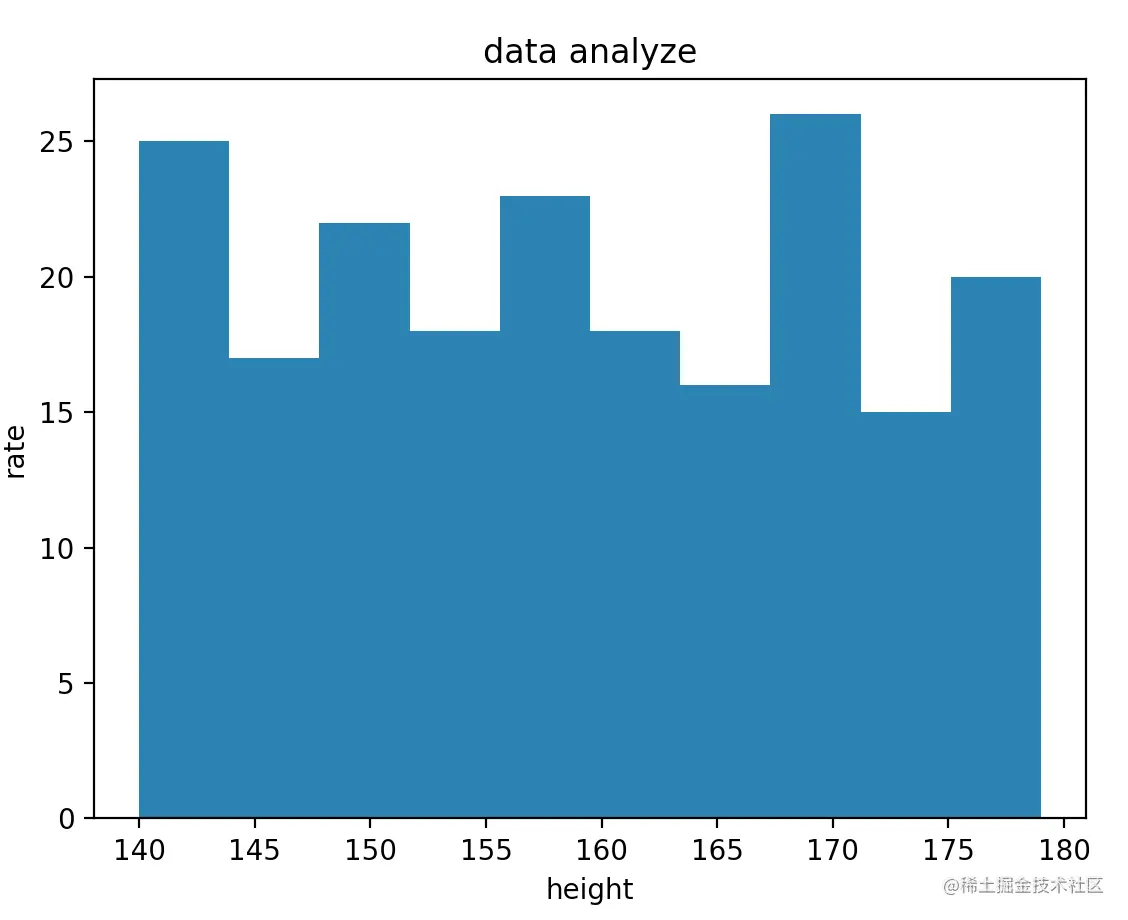# 2. Histogram properties

• ## Set the color

• Set the bar color keyword ：facecolor

• Set the color keyword of the border ：edgecolor

• Color selection value

• English words that use color, such as red "red", yellow "yellow"
• Use color abbreviations ： Red "r", Blue "b"
• Use rgb: Format (r,g,b), Value range ：0～1
• ## Set the number of long bars

• keyword ：bins
• optional , The default is 10
• ## Set transparency

• keyword ：alpha
• The default is 0, The value range is 0～1
• ## Set the style

• keyword ：histtype

• Value description

Property value explain
'bar' Column data side by side , The default value is
'barstacked' Column data overlaps side by side
'step' Columnar color does not fill
'stepfilled' Filled linear
• We add column shape to the first histogram without filling , The border color is red

``````plt.hist(x_value,bins=10,edgecolor="r",histtype="step")
Copy code ``````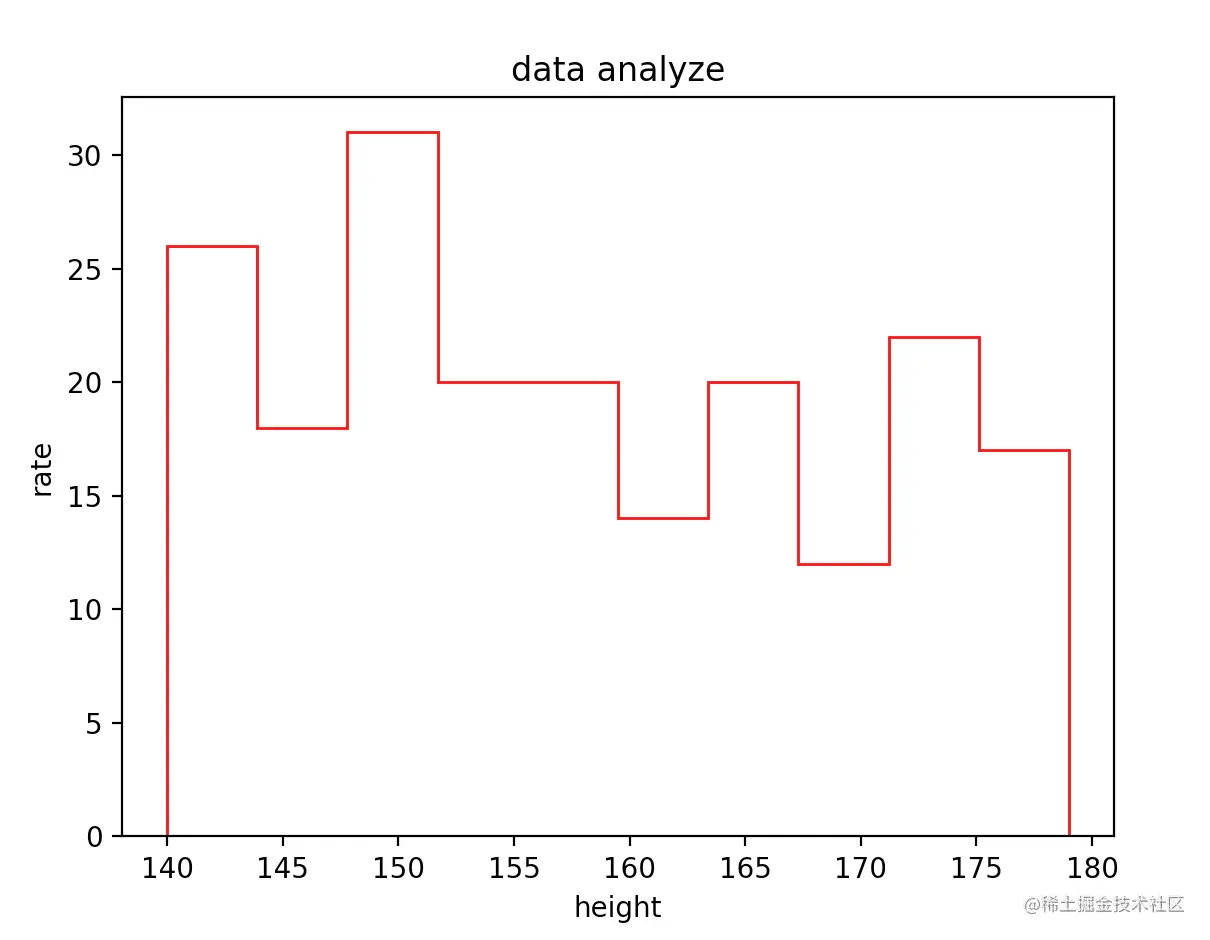# 3. Add a polyline histogram

In the histogram , We can also add a line chart , Help us check the data changes

• First, through pyplot.subplot() establish Axes object

• adopt Axes Object call hist() Method draw histogram , Return to the lower... Required by the line chart x,y data

• then Axes Object call plot() Draw line chart

• Let's modify the first section of the code

``````fig,ax = plt.subplots()

n,bins_num,pat = ax.hist(x_value,bins=10,alpha=0.75)

ax.plot(bins_num[:10],n,marker = 'o',color="yellowgreen",linestyle="--")
Copy code ``````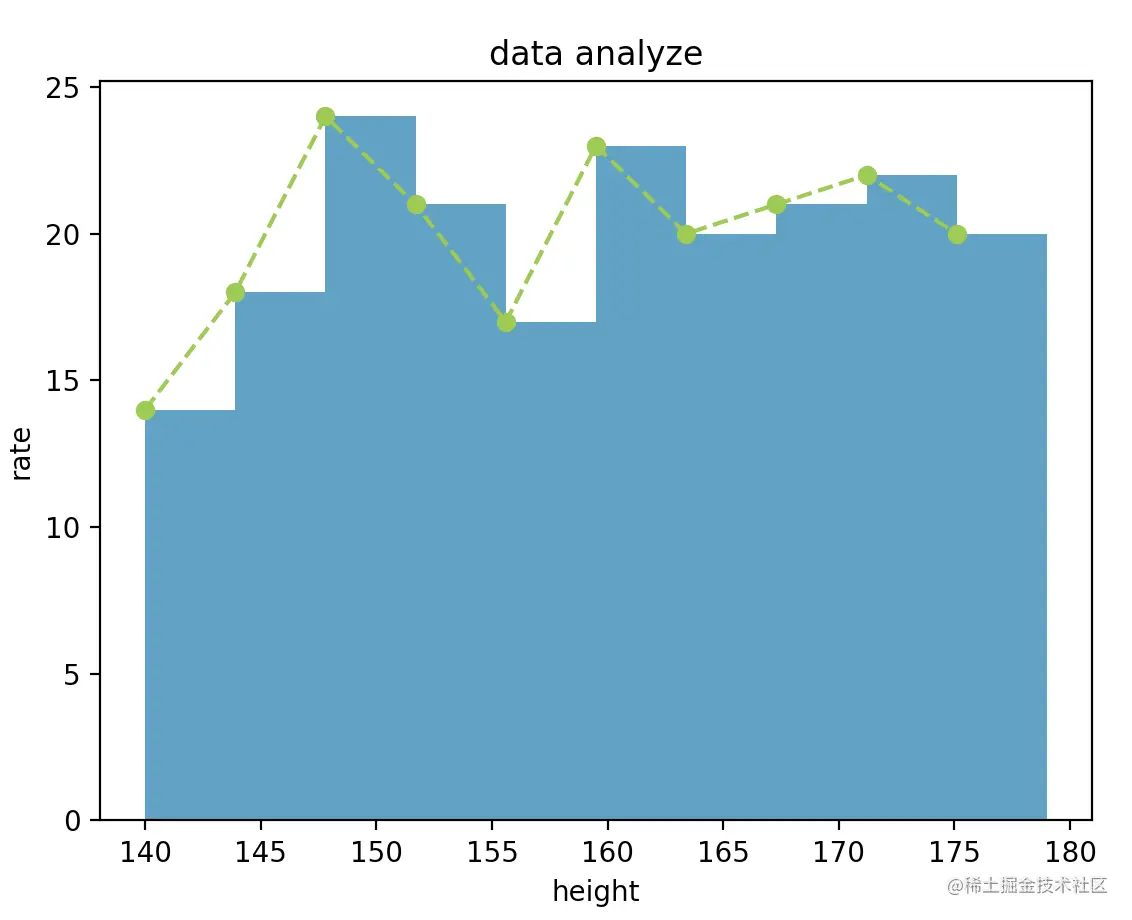# 4. Stacked Histogram

We sometimes, right? In the same data range , Compare the data collected by the two groups of different object groups

• Prepare two sets of data ：

``````import numpy as np

x_value = np.random.randint(140,180,200)
x2_value = np.random.randint(140,180,200)
Copy code ``````
• Histogram properties data： Pass in two sets of data in the form of a list

• Set histogram stacked: by True, Allow data coverage

``````plt.hist([x_value,x2_value],bins=10,stacked=True)
Copy code ``````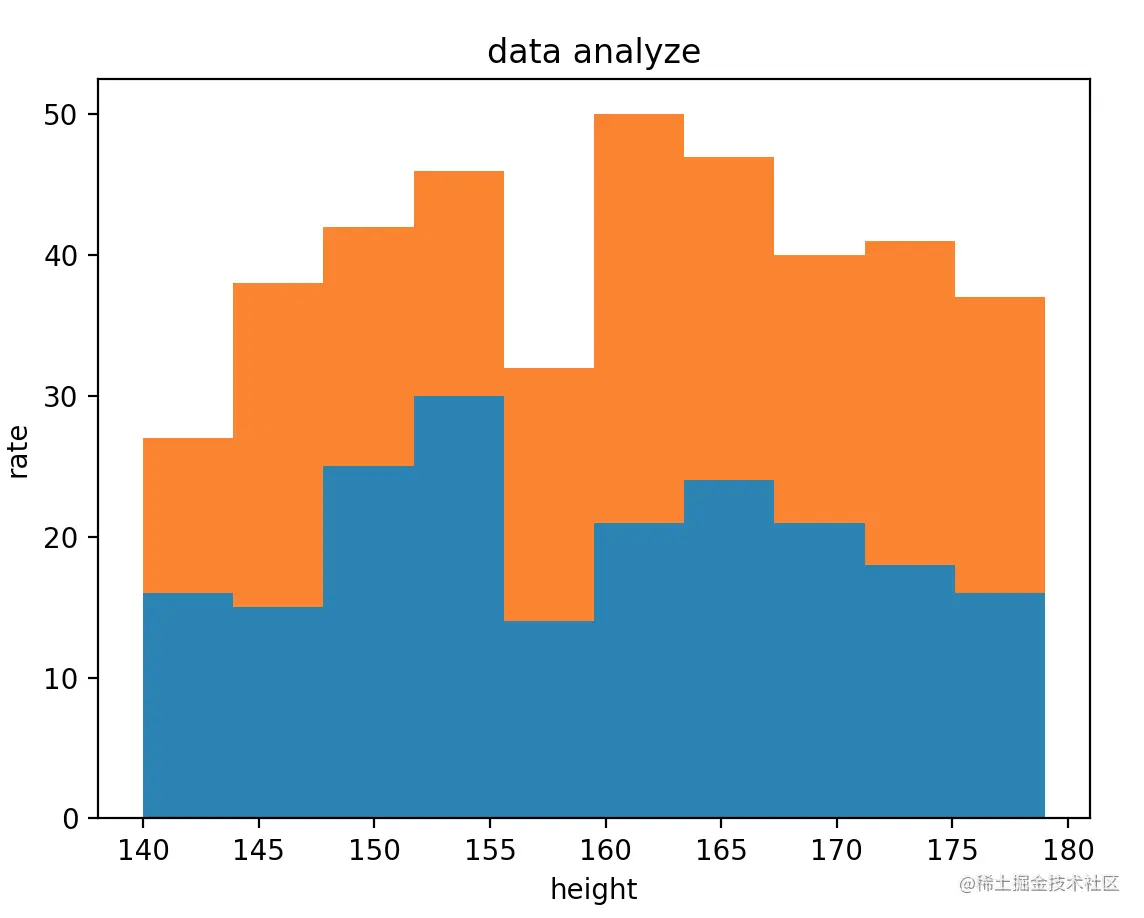# 5. Unequal histogram

The histograms we drew above are equidistant , We can specify a set of data to pass in bins attribute

• bins keyword ： Specifies the number of columns in the histogram

• After changing the above code , Let's see the effect

``````bin_num = [140,155,160,170,175,180]
plt.hist([x_value,x2_value],bins=bin_num,alpha=0.75,stacked=True)
Copy code ``````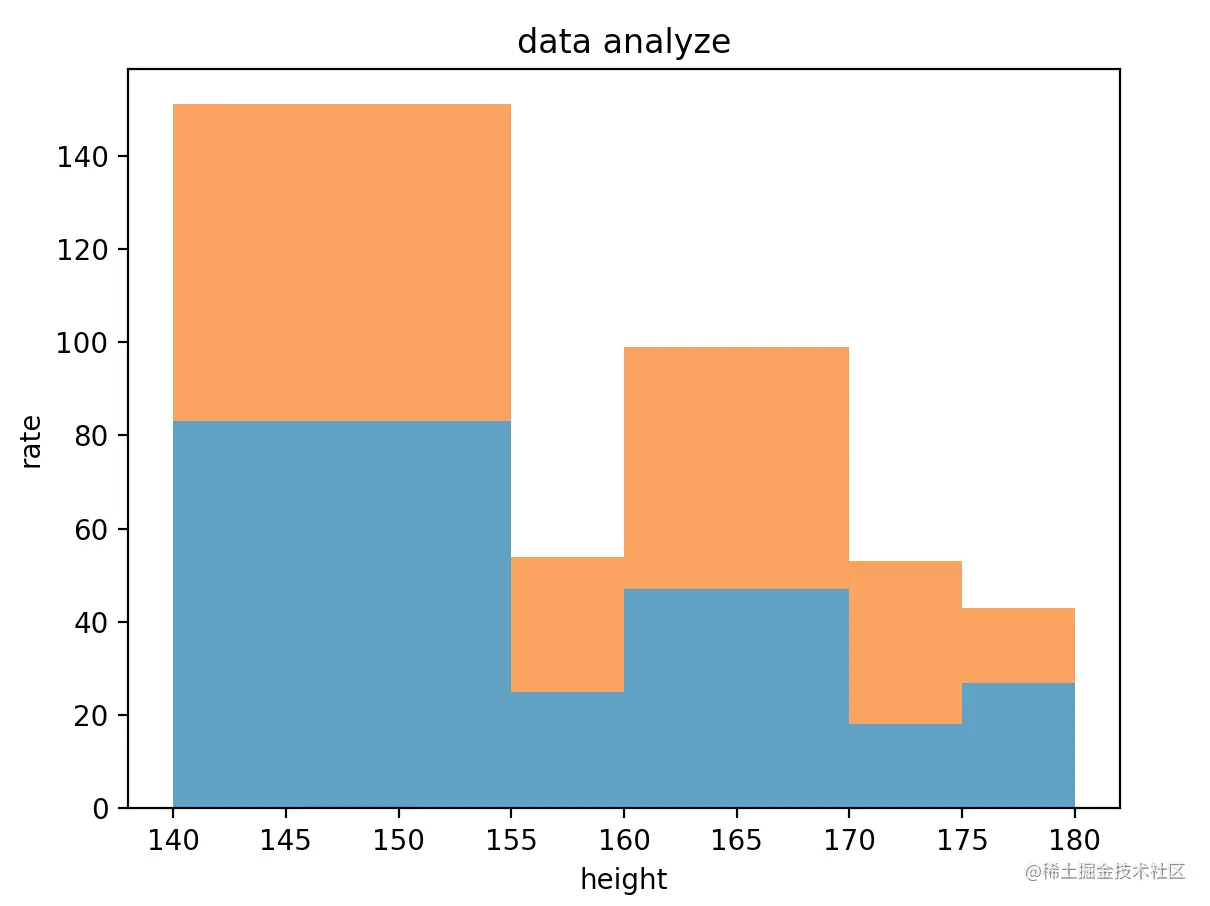# summary

In this issue , We are right. matplotlib In the module, learn the related attributes and methods of drawing various square icons in detail . When you need to view the frequency of data distribution , We can use hist() Method draw histogram , You can also add polylines to help you view

The above is the content of this issue , Welcome big guys to praise and comment , See you next time ～